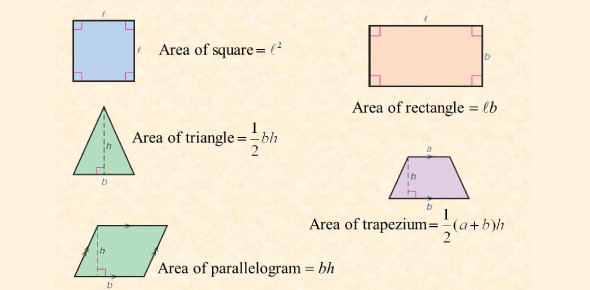# Area Of Plane Figures Quiz

15 Questions | Attempts: 4031
ShareSettingsAre you struggling with mathematics? Our ' Area Of Plane Figures Quiz' contains questions that can help you understand your mathematical concepts better. The questions are easy to understand and solve, so why not try? Try finding the area of triangles, parallelograms, trapezoids, etc., and check your progress via the scored card at the end. You have to attempt all the questions given carefully. If you encounter problems, refer to your books or teachers for advice. Practice makes a man perfect. Keep studying, and all the best!

• 1.
What do you call the measurement around the outside of a figure?
• A.

Perimeter

• B.

Area

• C.

Volume

• D.

Circumference

• 2.
What are you measuring the inside of a two dimensional figure such as a square, rectangle or triangle you are finding the...
• A.

Perimeter

• B.

Volume

• C.

Area

• D.

13 inches

• 3.
What is the proper way to label the area of a rectangle that has a length of 5 inches and a width of 4 inches?
• A.

20 inches

• B.

20 square inches

• C.

9 square inches

• 4.
When you measure the height of a figure the line must be straight ( not slanted).  It must be perpendicular ( at a 90 degree angle) to the base.
• A.

True

• B.

False

• 5.
The base of a figure...
• A.

Is always just the bottom only

• B.

May be the bottom as well as the top

• C.

Is always only the top

• D.

Is only on the side

• 6.
If a square measures 3 cm on each side, what is its area?
• A.

9 cm

• B.

12 cm

• C.

9 sq. cm

• D.

23 cm

• 7.
What is the area of a parallelogram that has a height of 7m and a base of 4 m?
• A.

14 square meters

• B.

11 square meters

• C.

28 square meters

• D.

15 square meters

• 8.
What is the area of a triangle that has a height of 9cm and a base of 4 cm?
• A.

18 sq cm

• B.

36 sq cm

• C.

13 sq cm

• D.

25 sq cm

• 9.
What is the area of a trapezoid that has a base of 2 inches, a base of 4  inches and a height of 3 inches?
• A.

6 sq. inches

• B.

24 sq. inches

• C.

9 sq inches

• D.

13 sq inches

• 10.
What is the area of a parallelogram that has a height of 21 m and a base of 9 m?
• A.

159 sq m

• B.

30 sq m

• C.

189 sq.m

• D.

100 sq. m

• 11.
What is the area of a trapezoid that has a base of 6 inches, a base of  10 inches and a height of 5 inches?
• A.

40 sq inches

• B.

21 sq inches

• C.

150 sq inches

• D.

25 inches

• 12.
What is the area of a triangle that has a height of 25 cm and a base of 20 cm?
• A.

420 cm

• B.

500 sq cm

• C.

250 sq.cm

• D.

298

• 13.
What is   22  x   34 ?
• A.

738

• B.

748

• C.

648

• 14.
What is  19 divided by 2?
• A.

9

• B.

9 and one half or 9.5

• C.

9.1

• D.

55

• 15.
What is  14  X   16 ?
• A.

204

• B.

144

• C.

224

• D.

544

## Related TopicsBack to top
×

Wait!
Here's an interesting quiz for you.